Characteristic Set Constraints

In order to formulate the constraints that will only license tree-shaped solved forms, we must first consider each individual case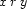for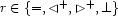. For each caseand its negation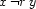, we will formulate characteristic constraints involving the set variables that we introduced above.

Let's consider the case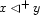for which a solution looks as shown below: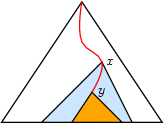For convenience, we define, for each variable, the additional set variables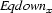and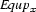as follows: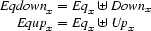We write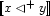for the constraint characteristic of caseand define it as follows: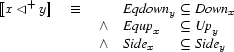I.e. all variables equal or beloware below, all variables equal or aboveare above, and all variables disjoint fromare also disjoint from. This illustrates how set constraints permit to succinctly express certain patterns of inference. Namelyprecisely expresses: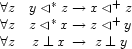The negation is somewhat simpler and states that no variable equal tois above, and no variable equal tois below. Remember that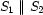expresses that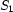and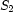are disjoint.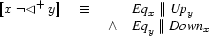We can define the other cases similarly. Thus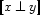: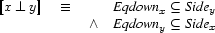and its negation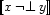: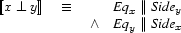For the case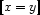we first introduce notation. We write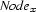for the tuple defined as follows: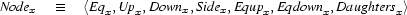where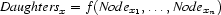when the constraint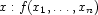occurs in(more about this when presenting the problem-specific constraints). Now we can simply defineas: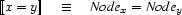and its negation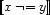as: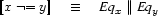Denys Duchier
Version 1.2.0 (20010221)